# Python中用PyTorch机器学习神经网络分类预测银行客户流失模型

## 分类问题属于机器学习问题的类别，其中给定一组特征，任务是预测离散值。

`` \$ pip install pytorch ``

# 数据集

`` dataset = pd.read_csv(r'E:Datasetscustomer_data.csv') ``

`` dataset.shape ``

`` (10000, 14) ``

LSTM神经网络架构和原理及其在Python中的预测应用

`` dataset.head() ``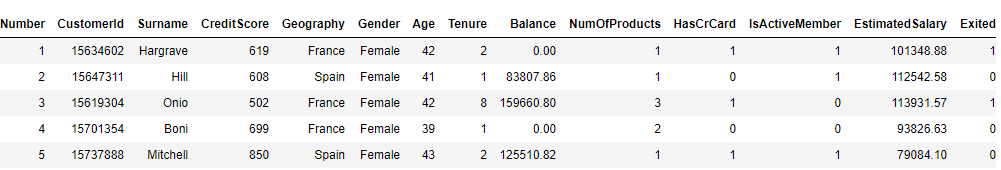# 探索性数据分析

`````` fig_size = plt.rcParams["figure.figsize"]
fig_size = 10
fig_size = 8
plt.rcParams["figure.figsize"] = fig_size ``````

`` dataset.Exited.value_counts().plot(kind='pie', autopct='%1.0f%%', colors=['skyblue', 'orange'], explode=(0.05, 0.05)) ``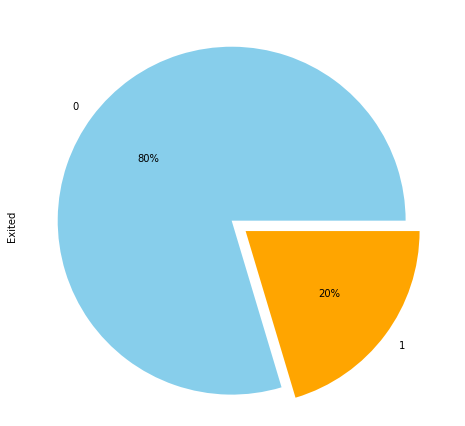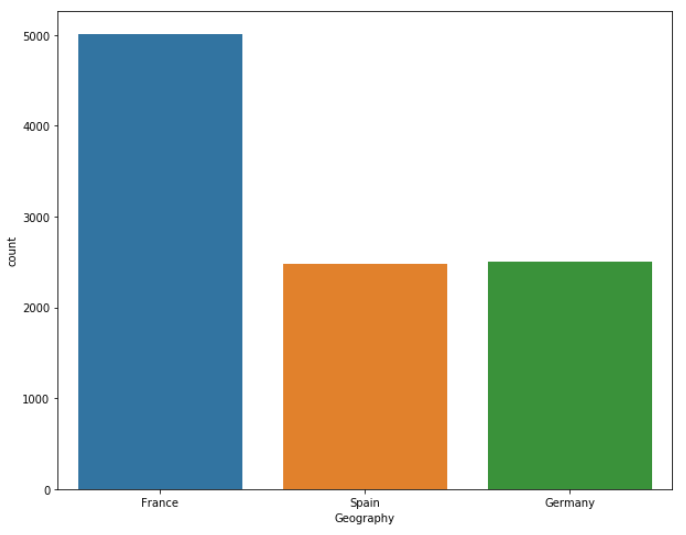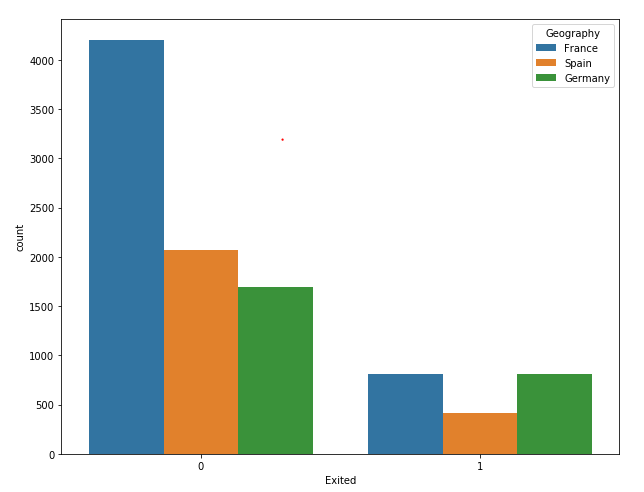# 数据预处理

``Index(['RowNumber', 'CustomerId', 'Surname', 'CreditScore', 'Geography', 'Gender', 'Age', 'Tenure', 'Balance', 'NumOfProducts', 'HasCrCard', 'IsActiveMember', 'EstimatedSalary', 'Exited'], dtype='object')``

# 用R语言实现神经网络预测股票实例

`` numerical_columns = ['CreditScore', 'Age', 'Tenure', 'Balance', 'NumOfProducts', 'EstimatedSalary'] ``

`````` RowNumber            int64
CustomerId           int64
Surname             object
CreditScore          int64
Geography           object
Gender              object
Age                  int64
Tenure               int64
Balance            float64
NumOfProducts        int64
HasCrCard            int64
IsActiveMember       int64
EstimatedSalary    float64
Exited               int64
dtype: object ``````

### 随时关注您喜欢的主题

`````` RowNumber             int64
CustomerId            int64
Surname              object
CreditScore           int64
Geography          category
Gender             category
Age                   int64
Tenure                int64
Balance             float64
NumOfProducts         int64
HasCrCard          category
IsActiveMember     category
EstimatedSalary     float64
Exited                int64
dtype: object ``````

``Index(['France', 'Germany', 'Spain'], dtype='object') ``

`````` 0    France
1     Spain
2    France
3    France
4     Spain
Name: Geography, dtype: category
Categories (3, object): [France, Germany, Spain] ``````

`````` 0    0
1    2
2    0
3    0
4    2
dtype: int8 ``````

# 将分类列与数字列分开的基本目的是，可以将数字列中的值直接输入到神经网络中。但是，必须首先将类别列的值转换为数字类型。分类列中的值的编码部分地解决了分类列的数值转换的任务。

`````` geo = dataset['Geography'].cat.codes.values
... ``````

`````` array([[0, 0, 1, 1],
[2, 0, 0, 1],
[0, 0, 1, 0],
[0, 0, 0, 0],
[2, 0, 1, 1],
[2, 1, 1, 0],
[0, 1, 1, 1],
[1, 0, 1, 0],
[0, 1, 0, 1],
[0, 1, 1, 1]], dtype=int8) ``````

`````` tensor([[0, 0, 1, 1],
[2, 0, 0, 1],
[0, 0, 1, 0],
[0, 0, 0, 0],
[2, 0, 1, 1],
[2, 1, 1, 0],
[0, 1, 1, 1],
[1, 0, 1, 0],
[0, 1, 0, 1],
[0, 1, 1, 1]]) ``````

`````` numerical_data = np.stack([dataset[col].values for col in numerical_columns], 1)
... ``````

# 在输出中，您可以看到前五行，其中包含我们数据集中六个数字列的值。最后一步是将输出的numpy数组转换为`tensor`对象。输出：

`````` tensor([[6.1900e+02, 4.2000e+01, 2.0000e+00, 0.0000e+00, 1.0000e+00, 1.0135e+05],
[6.0800e+02, 4.1000e+01, 1.0000e+00, 8.3808e+04, 1.0000e+00, 1.1254e+05],
[5.0200e+02, 4.2000e+01, 8.0000e+00, 1.5966e+05, 3.0000e+00, 1.1393e+05],
[6.9900e+02, 3.9000e+01, 1.0000e+00, 0.0000e+00, 2.0000e+00, 9.3827e+04],
[8.5000e+02, 4.3000e+01, 2.0000e+00, 1.2551e+05, 1.0000e+00, 7.9084e+04]]) ``````

`` tensor([1, 0, 1, 0, 0]) ``

`````` torch.Size([10000, 4])
torch.Size([10000, 6])
torch.Size() ``````

`````` categorical_column_sizes = [len(dataset[column].cat.categories) for column in categorical_columns]
``````

`` [(3, 2), (2, 1), (2, 1), (2, 1)] ``

`````` total_records = 10000
``````

`````` print(len(categorical_train_data))
print(len(numerical_train_data))
print(len(train_outputs))

print(len(categorical_test_data))
print(len(numerical_test_data))
print(len(test_outputs)) ``````

`````` 8000
8000
8000
2000
2000
2000``````

# 创建预测模型

`````` class Model(nn.Module):

def __init__(self, embedding_size, num_numerical_cols, output_size, layers, p=0.4):
super().__init__()
self.all_embeddings = nn.ModuleList([nn.Embedding(ni, nf) for ni, nf in embedding_size])
self.embedding_dropout = nn.Dropout(p)
self.batch_norm_num = nn.BatchNorm1d(num_numerical_cols)

return x ``````

• `Linear`：用于计算输入和权重矩阵之间的点积
• `ReLu`：用作激活函数
• `BatchNorm1d`：用于对数字列应用批量归一化
• `Dropout`：用于避免过拟合

``embeddings = []``

``x_numerical = self.batch_norm_num(x_numerical)``

# 训练模型

`` print(model) ``

`````` Model(
(all_embeddings): ModuleList(
)
) ``````

`` loss_function = nn.CrossEntropyLoss() ``

`````` epochs = 300
aggregated_losses = []

for i in range(epochs):

print(f'epoch: {i:3} loss: {single_loss.item():10.10f}') ``````

``````epoch: 1 loss: 0.71847951
epoch: 26 loss: 0.57145703
epoch: 51 loss: 0.48110831
epoch: 76 loss: 0.42529839
epoch: 101 loss: 0.39972275
epoch: 126 loss: 0.37837571
epoch: 151 loss: 0.37133673
epoch: 176 loss: 0.36773482
epoch: 201 loss: 0.36305946
epoch: 226 loss: 0.36079505
epoch: 251 loss: 0.35350436
epoch: 276 loss: 0.35540250
epoch: 300 loss: 0.3465710580``````

``````plt.plot(range(epochs), aggregated_losses)
plt.ylabel('Loss')
plt.xlabel('epoch')``````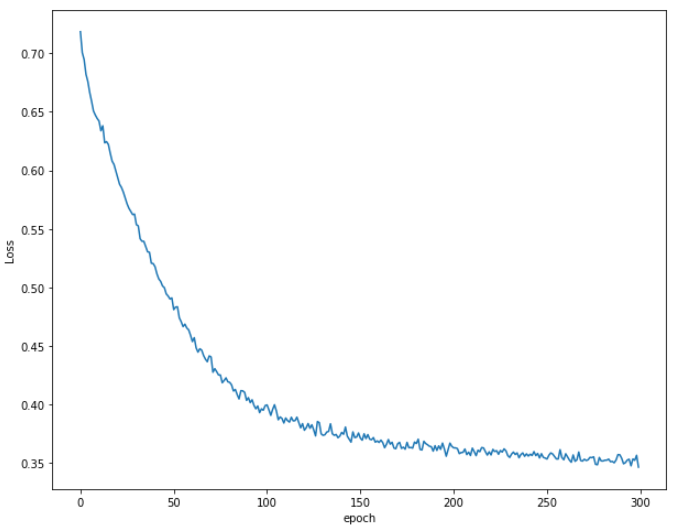# 做出预测

`````` with torch.no_grad():
``````

`` Loss: 0.36855841 ``

`` print(y_val[:5]) ``

`````` tensor([[ 1.2045, -1.3857],
[ 1.3911, -1.5957],
[ 1.2781, -1.3598],
[ 0.6261, -0.5429],
[ 2.5430, -1.9991]]) ``````

`` y_val = np.argmax(y_val, axis=1) ``

`` print(y_val[:5]) ``

`` tensor([0, 0, 0, 0, 0]) ``

``from sklearn.metrics import classification_report, confusion_matrix, accuracy_score``
``````print(confusion_matrix(test_outputs,y_val))
print(classification_report(test_outputs,y_val))
print(accuracy_score(test_outputs, y_val))``````

``[[1527 83][ 224 166]]``
``````          precision    recall  f1-score   support

0       0.87      0.95      0.91      1610
1       0.67      0.43      0.52       390
``````
``micro avg 0.85 0.85 0.85 2000macro avg 0.77 0.69 0.71 2000weighted avg 0.83 0.85 0.83 2000``
``0.8465``

# 结论

Kaizong Ye拓端研究室（TRL）的研究员。

​非常感谢您阅读本文，如需帮助请联系我们！QQ在线咨询

15121130882

0571-63341498

## 关注有关新文章的微信公众号

This will close in 0 seconds